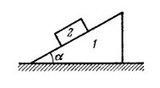# Pseudo Force ~ (1 Viewer)

### Users Who Are Viewing This Thread (Users: 0, Guests: 1)

#### sArGe99

1. The problem statement, all variables and given/known data

Prism 1 with bar 2 of mass m placed on it gets a horizontal acceleration $$\omega$$ directed to the left. At what maximum value of this acceleration $$\omega$$ will the bar be still stationary relative to the prism, if the coefficient of friction between them $$k < cot\alpha$$2. Relevant equations

$$mg sin \alpha - m \omega cos \alpha$$ = Force trying to move the body along the prism.
k ($$m\omega sin \alpha + mg cos \alpha$$) = Frictional force.

3. The attempt at a solution
Since the prism is accelerating to the left, the bar experiences a pseudo force $$m\omega$$ directed to the right. Resolving it has components
$$m \omega cos \alpha$$ opposed to $$mg sin \alpha$$ and the component $$m\omega sin \alpha$$ acting along the direction of $$mg cos \alpha$$

At equilibrium, the frictional force equals the force which acts along the prism.
Equating, $$mg sin \alpha - m\omega cos\alpha$$ = k ($$m\omega sin \alpha + mg cos \alpha$$)
Further simplifying,

$$\omega = \frac {g (sin \alpha - k cos \alpha)}{cos \alpha + k sin \alpha}$$

This is not the correct answer. Can you please point out where I've made a mistake?

#### Doc Al

Mentor
At equilibrium, the frictional force equals the force which acts along the prism.
Equating, $$mg sin \alpha - m\omega cos\alpha$$ = k ($$m\omega sin \alpha + mg cos \alpha$$)
Looks like you made a sign error. Which way does the friction force act? Which direction are you calling positive?

(I'd use ΣF = 0 parallel to the wedge, coupled with a consistent sign convention.)

#### hsarp71

the maximum value of k is cot(alpha). So have you tried putting that in?

#### sArGe99

Looks like you made a sign error. Which way does the friction force act? Which direction are you calling positive?

(I'd use ΣF = 0 parallel to the wedge, coupled with a consistent sign convention.)
Oh. Thanks for that correction. I've got the right answer now.I reckon I can use the sign system in any direction as long as I put the signs correctly?

#### sArGe99

Its given $$k < cot\alpha$$. What is the importance of this data in this question? I got the correct answer although I didn't have this point in mind. So I'm thinking why its given..

Last edited by a moderator:

#### Doc Al

Mentor
I reckon I can use the sign system in any direction as long as I put the signs correctly?
Absolutely.
Its given $$k < cot\alpha$$. What is the importance of this data in this question? I got the correct answer although I didn't have this point in mind. So I'm thinking why its given..
Consider the sign of your expression for the acceleration if that condition were not met.#### sArGe99

Sign of the expression for acceleration? I don't notice any particular change when $$k < cot\alpha$$!!Where am I going wrong?

#### Doc Al

Mentor
Sign of the expression for acceleration? I don't notice any particular change when $$k < cot\alpha$$!!Where am I going wrong?
Take a look at your (corrected) expression. What happens to the sign of the denominator when k > cot α ?

Last edited:

#### sArGe99

The denominator turns out to be zero when $$k= cot\alpha$$. :tongue:
Thanks for the help.

Last edited:

#### sArGe99

I see that the tendency of the body 2 is to move up. Why is it like that, usually it always tends to move down, right?

#### Doc Al

Mentor
I see that the tendency of the body 2 is to move up. Why is it like that, usually it always tends to move down, right?
The block would tend to move down the prism, if the prism weren't accelerating towards it. That changes things by introducing additional forces.

### The Physics Forums Way

We Value Quality
• Topics based on mainstream science
• Proper English grammar and spelling
We Value Civility
• Positive and compassionate attitudes
• Patience while debating
We Value Productivity
• Disciplined to remain on-topic
• Recognition of own weaknesses
• Solo and co-op problem solving